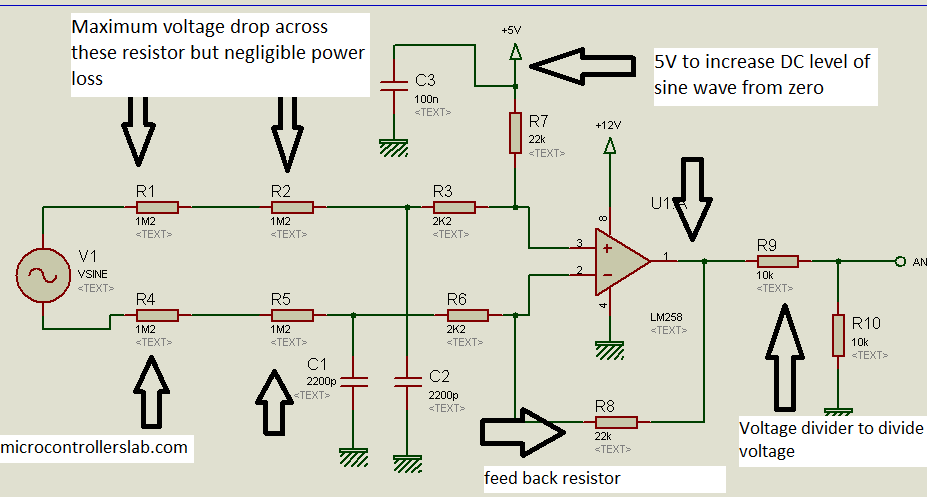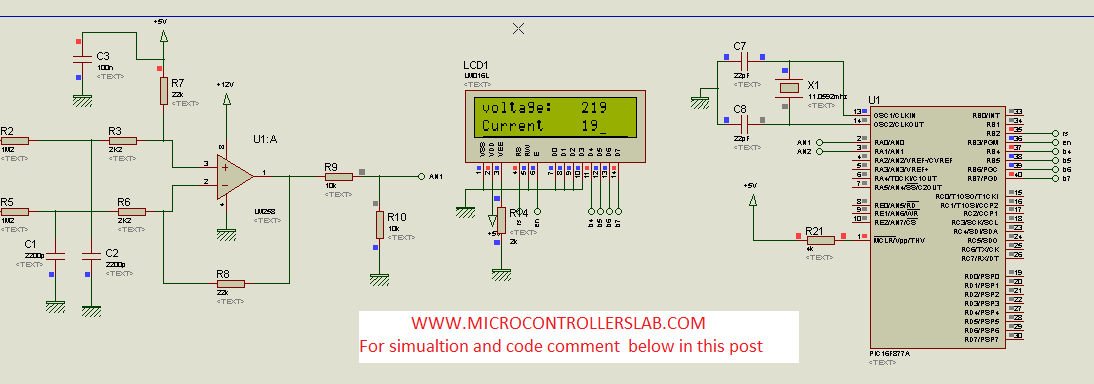# AC Voltage measurement using PIC16F877A microcontroller

AC voltage measurement using pic microcontroller and op-amp.You have came across many online tutorials  on various websites about voltage measurement using different microcontrollers. But all these tutorials are about measurement of low  DC voltage.In this project, you will learn how to measure high AC voltage using PIC16f877A micrcontroller.

## Alternating voltage measurement :

To measure 220V AC, you need to step down this voltage. Because microcontrollers can’t measure voltage greater than 5V. If you give voltage more than 5V to the analog input of microcontroller, it will get damage permanently. To assure protection of microcontroller, you will need to step down 220 volt AC into AC  voltage whose peak value should be less than 5V. For example , 220V AV mean RMS voltage and its peak value is equal to 311 volt. similarly you have to step down high AC voltage of in such a way that its peak value should not be greater than 5 volt.

There are two methods to step down 220 alternating voltage into low alternating voltage which peak value should not be greater than 5 volt.

• Potential Transformer ( All Electrical Engineering students must know about P.T and its use)
• Difference amplifier  ( I will discuss difference amplifier method  in this project.)
READ  DATA LOGGER measures and stores voltage using PIC16F876

Potential Transformer can also be used to step down 220 Alternation voltage.But why you want to spend more money? when you can do this with the help of cheap operational amplifiers and few resistors. Difference amplifier method is more economical than potential Transformer when you want to step down voltage less than 400 volt AC.

NOTE: Difference amplifier method is economical for voltage measurement less than 400 volt. Because above 400 volt, this method become expensive than potential Transformer. There are reasons behind it. I am not going to discuss reasons here.This method is suitable for final year students who want  to measure Alternating voltage and current.

### Difference Amplifier:

Difference amplifier is used to amplify voltage from two voltage levels.In case of Alternating voltage we have two voltage level one is positive with respect to neutral and other is negative with respect to neutral. I will recommend you to Google about difference amplifier and its use.

you can adjust the gain of difference amplifier according to our requirement by selecting proper  values of resistors.In this project gain is equal to :

Gain=  R8/(R1+ R2+ R3)  ;

In Alternating voltage case second voltage level is zero. Because during positive cycle and negative cycle other side is considered zero or neutral. So output voltage will be

vout = gain * Vinput;Difference amplifier to step down voltage

In Above picture resistor R1, R2, R3 R4, and R5 have high values which do not allow high voltage to appear across op-amp. Because high input resistors are used that’s why micro ampere current will low and this way power loss will be in mili watts. According to difference amplifier gain formula our gain will be:

READ  Understanding Timers in PIC Microcontroller with LED Blinking Sequence

gain=   (22K)/( 1.2M + 1.2M + 2.2K) = 0.0091

NOTE:  you must make calculation according to peak value of sine wave. Because peak voltage is the maximum voltage input to micrcontroller analog pin.So with a .0091 gain, with respect to peak voltage of sine wave output voltage from op-amp is :

Vout = .0091 * 311 = 2.8301 volt (peak output voltage)

As you see above figure we have connected other terminal of R7 to 5 volt instead of  ground as we do while using difference amplifier in many applications. R7 resistor is used to increase DC voltage level op-amp output. Sine wave have zero DC voltage level and  negative voltage cycle. Microcontroller can not read negative voltage.So we increase the DC level of sine wave by 5 volt.In this way negative voltage will not appear across  microntroller. Now output peak voltage from op-amp is 5 + 2.8301 = 7.8301 volt. But as I have mentioned , microcontrollers can not measure voltage greater than 5 volt.So as shown in above figure we have used voltage divider to divide voltage by 2.Hence output voltage is:

Vout = 7.8301/2 =  3.90155;

Capacitors C1, C2, and C3 are used to filter harmonics from input voltage and to provide protection to micrcontroller from harmonics.Now AN pin can be connected to microcontroller analog pin to measure voltage easily.

### Video lecture on AC voltmeter design

To know about how to measure analog voltage using analog module of PIC16F877A microcontroller go through PIC microcontrollers tutorials..

PIC MICROCONTROLLERS TUTORIALS

### COMPLETE CIRCUIT DIAGRAM:

To know about LCD interfacing with PIC micronctrollers, go through pic microcontrollers        tutorials

READ  Mechanically scanned RS232 display (with dynamic speed)

PIC MICROCONTROLLERS TUTORIALS

Complete circuit diagram:If you want to get complete code and circuit diagram comment on this  with your email address.

### AC voltage measurement code

Code for this project is written using Mikro C.To download code for AC voltage measurement click on link below :  [sociallocker]

```sbit LCD_RS at RB2_bit;
sbit LCD_EN at RB3_bit;
sbit LCD_D4 at RB4_bit;
sbit LCD_D5 at RB5_bit;
sbit LCD_D6 at RB6_bit;
sbit LCD_D7 at RB7_bit;
sbit LCD_RS_Direction at TRISB2_bit;
sbit LCD_EN_Direction at TRISB3_bit;
sbit LCD_D4_Direction at TRISB4_bit;
sbit LCD_D5_Direction at TRISB5_bit;
sbit LCD_D6_Direction at TRISB6_bit;
sbit LCD_D7_Direction at TRISB7_bit;
float v;
char txt;
char txt1;
{
float max;
int i;
int t;
for(i=0; i<=39; i++)
{
v =v*(10.0/1023.0);
v=(v-5.0);
t[i]=v*110.1909091;
}
max=t;
for(i=0; i<=39; i++)
{
if(max<t[i])
max=t[i];
}
max=max*.707106781;
intToStr(max, txt);
Lcd_out(1,9,txt);
delay_ms(1000);
}
void main()
{
Lcd_Init(); // Initialize LCD

JLCPCB – Prototype 10 PCBs for \$2 (For Any Color)
China’s Largest PCB Prototype Enterprise, 600,000+ Customers & 10,000+ Online Orders Daily
See Why JLCPCB Is So Popular: https://jlcpcb.comCurrent Project / Post can also be found using:

ac volt metre using PIC
code for PIC ac volt meter
pic assemly code for ac voltage measurements pdf
ac voltage measurement using pic

```